# Factor To Fuzzy set theory (Statistics)

### Factor:

A term used in a variety of ways in statistics, but most commonly to refer to a categorical variable, with a small number of levels, under investigation in an experiment as a possible source of variation. Essentially simply a categorical explanatory variable.

### Factor analysis:

A procedure that postulates that the correlations or covariances between a set of observed variables, x’ = [x1 , X2; • ••, Xq], arise from the relationship of these variables to a small number of underlying, unobservable, latent variables, usually known as the common factors, f = [f1, f2, •••, fk], where k < q. Explicitly the model used is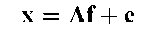where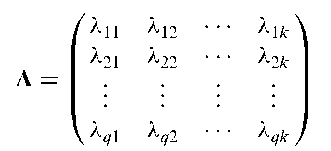contains the regression coefficients (usually known in this context as factor loadings) of the observed variables on the common factors. The matrix, A, is known as the loading matrix. The elements of the vector e are known as specific variates. Assuming that the common factors are uncorrelated, in standardized form and also uncorre-lated with the specific variates, the model implies that the variance-covariance matrix of the observed variables, D, is of the form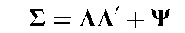where ) is a diagonal matrix containing the variances of the specific variates. A number of approaches are used to estimate the parameters in the model, i.e. the elements of A and ), including principal factor analysis and maximum likelihood estimation. After the initial estimation phase an attempt is generally made to simplify the often difficult task of interpreting the derived factors using a process known as factor rotation. In general the aim is to produce a solution having what is known as simple structure, i.e. each common factor affects only a small number of the observed variables. Although based on a well-defined model the method is, in its initial stages at least, essentially exploratory and such exploratory factor analysis needs to be carefully differentiated from confirmatory factor analysis in which a prespecified set of common factors with some variables constrained to have zero loadings is tested for consistency with the correlations of the observed variables. See also structural equation models and principal components analysis. [MV2 Chapter 12.]

### Factorial designs:

Designs which allow two or more questions to be addressed in an investigation. The simplest factorial design is one in which each of two treatments or interventions are either present or absent, so that subjects are divided into four groups; those receiving neither treatment, those having only the first treatment, those having only the second treatment and those receiving both treatments. Such designs enable possible interactions between factors to be investigated. A very important special case of a factorial design is that where each of k factors of interest has only two levels; these are usually known as 2k factorial designs. Particularly important in industrial applications of response surface methodology. A single replicate of a 2k design is sometimes called an unreplicated factorial.

### Factorial moment generating function:

A function of a variable t which, when expanded formally as a power series in t, yields the factorial moments as coefficients of the respective powers. If P(t) is the probability generating function of a discrete random variable, the factorial moment generating function is simply P(1 +1).

### Factorial moments:

A type of moment used almost entirely for discrete random variables, or occasionally for continuous distributions grouped into intervals. For a discrete random variable, X, taking values 0,1, 2,…, 1, the jth factorial moment about the origin, f j is defined asBy direct expansion it is seen that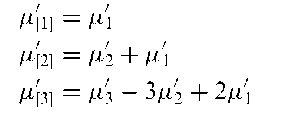### Factorization theorem:

A theorem relating the structure of the likelihood to the concept of a sufficient statistic. Formally a necessary and sufficient condition that a statistic S be sufficient for a parameter 0 is that the likelihood, 1(0; y) can be expressed in the form; 1(0; y) = m1(S,0)m2(y) For example, if Y1, Y2,…, Yn are independent random variables from a Poisson distribution with mean f, the likelihood is given by;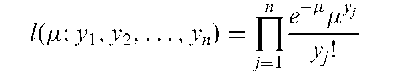which can be factorized into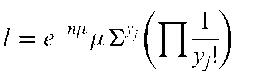Consequently S = J]yj is a sufficient statistic for f.

### Factor rotation:

Usually the final stage of a factor analysis in which the factors derived initially are transformed to make their interpretation simpler. In general the aim of the process is to make the common factors more clearly defined by increasing the size of large factor loadings and decreasing the size of those that are small. Bipolar factors are generally split into two separate parts, one corresponding to those variables with positive loadings and the other to those variables with negative loadings. Rotated factors can be constrained to be orthogonal but may also be allowed to be correlated or oblique if this aids in simplifying the resulting solution. See also varimax rotation.

### Factor scores:

Because the model used in factor analysis has more unknowns than the number of observed variables (q), the scores on the k common factors can only be estimated, unlike the scores from a principal component analysis which can be obtained directly. The most common method of deriving factor scores is least squares estimation.

### Factor sparsity:

A term used to describe the belief that in many industrial experiments to study large numbers of factors in small numbers of runs, only a few of the many factors studied will have major effects. [Technometrics, 1996, 38, 303-13.]

### Failure record data:

Data in which time to failure of an item and an associated set of explanatory variables are observed only for those items that fail in some prespecified warranty period (0, T0]. For all other items it is known only that T, > T0, and for these items no covariates are observed.

### Failure time:

Synonym for survival time.

### Fair game:

A game in which the entry cost or stake equals the expected gain. In a sequence of such games between two opponents the one with the larger capital has the greater chance of ruining his opponent. See also random walk.

### False discovery rate (FDR):

An approach to controlling the error rate in an exploratory analysis where large numbers of hypotheses are tested, but where the strict control that is provided by multiple comparison procedures controlling the familywise error rate is not required. Suppose m hypotheses are to be tested, of which m0 relate to cases where the null hypothesis is true and the remaining m — m0 relate to cases where the alternative hypothesis is true. The FDR is defined as the expected proportion of incorrectly rejected null hypotheses. Explicitly the FDR is given by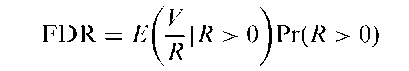where V represents the number of true null hypotheses that are rejected and R is the total number of rejected hypotheses. Procedures that exercise control over the FDR guarantee that FDR< a, for some fixed value of a.

### False-negative rate:

The proportion of cases in which a diagnostic test indicates disease absent in patients who have the disease. See also false-positive rate.

### False-positive rate:

The proportion of cases in which a diagnostic test indicates disease present in disease-free patients. See also false-negative rate.

### Familial correlations:

The correlation in some phenotypic trait between genetically related individuals. The magnitude of the correlation is dependent both on the heritability of the trait and on the degree of relatedness between the individuals.

### Familywise error rate:

The probability of making any error in a given family of inferences.

A term sometimes applied to a model for explaining differences found between naturally occurring groups that are greater than those observed on some earlier occasion; under this model this effect is assumed to arise because individuals who are less unwell, less impaired, etc., and thus score higher initially, may have greater capacity for change or improvement over time.

### Farr, William (1807-1883):

Born in Kenley, Shropshire, Farr studied medicine in England from 1826 to 1828 and then in Paris in 1829. It was while in Paris that he became interested in medical statistics. Farr became the founder of the English national system of vital statistics. On the basis of national statistics he compiled life tables to be used in actuarial calculations. Farr was elected a Fellow of the Royal Society in 1855 and was President of the Royal Statistical Society from 1871 to 1873. He died on 14 April 1883.

### Fast Fourier transformation (FFT):

A method of calculating the Fourier transform of a set of observations x0, x1,…, xn_1, i.e. calculating d(wp) given by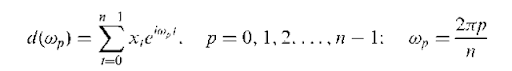The number of computations required to calculate {d(up)} is n2 which can be very large. The FFT reduces the number of computations to O(n log2 n), and operates in the following way.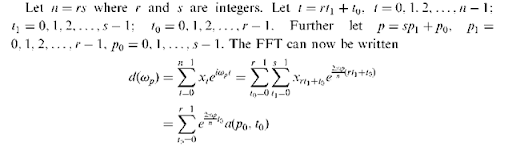where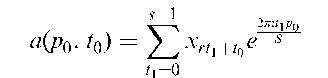Calculation of a(p0, t0) requires only s2 operations, and d(wp) only rs2. The evaluation of d(!p) reduces to the evaluation of a(p0, t0) which is itself a Fourier transform. Following the same procedure, the computation of a(p0, t0) can be reduced in a similar way. The procedures can be repeated until a single term is reached.

### Fatigue models:

Models for data obtained from observations of the failure of materials, principally metals, which occurs after an extended period of service time. See also variable-stress accelerated life testing trials. [Methods for Statistical Analysis of Reliability and Life Data, 1974, N.R. Mann, R.E. Schafer and N.D. Singpurwalla, Wiley, New York.]

### F-distribution:

The probability distribution of the ratio of two independent random variables, each having a chi-squared distribution, divided by their respective degrees of freedom. Widely used to assign P-values to mean square ratios in the analysis of variance. The form of the distribution function is that of a beta distribution with a = vj/2 and p = v2/2 where v1 and v2 are the degrees of freedom of the numerator and denominator chi-squared variables, respectively. [STD Chapter 16.]

### Feasibility study:

Essentially a synonym for pilot study.

### Feller, William (1906-1970):

Born in Zagreb, Croatia, Feller was educated first at the University of Zagreb and then at the University of Gottingen from where he received a Ph.D. degree in 1926. After spending some time in Copenhagen and Stockholm, he moved to Providence, Rhode Island in 1939. Feller was appointed professor at Cornell University in 1945 and then in 1950 became Eugene Higgins Professor of Mathematics at Princeton University, a position he held until his death on 14 January 1970. Made significant contributions to probability theory and wrote a very popular two-volume text An Introduction to Probability Theory and its Applications. Feller was awarded a National Medal for Science in 1969.

### FFT:

Abbreviation for fast Fourier transform.

### Fibonacci distribution:

The probability distribution of the number of observations needed to obtain a run of two successes in a series of Bernoulli trials with probability of success equal to a half. Given by

Pr(X = x) = 2-xF2tX-2 where the F2 xs are Fibonacci numbers. [Fibonacci Quarterly, 1973, 11, 517-22.]

### Fiducial inference:

A problematic and enigmatic theory of inference introduced by Fisher, which extracts a probability distribution for a parameter on the basis of the data without having first assigned the parameter any prior distribution. The outcome of such an inference is not the acceptance or rejection of a hypothesis, not a decision, but a probability distribution of a special kind.

### Field plot:

A term used in agricultural field experiments for the piece of experimental material to which one treatment is applied.

### Fieller’s theorem:

A general result that enables confidence intervals to be calculated for the ratio of two random variables with normal distributions.

### File drawer problem:

The problem that studies are not uniformly likely to be published in scientific journals. There is evidence that statistical significance is a major determining factor of publication since some researchers may not submit a nonsignificant results for publication and editors may not publish nonsignificant results even if they are submitted. A particular concern for the application of meta-analysis when the data come solely from the published scientific literature.

### Final-state data:

A term often applied to data collected after the end of an outbreak of a disease and consisting of observations of whether or not each individual member of a household was ever infected at any time during the outbreak.

### Finite mixture distribution:

A probability distribution that is a linear function of a number of component probability distributions. Such distributions are used to model populations thought to contain relatively distinct groups of observations. An early example of the application of such a distribution was that of Pearson in 1894 who applied the following mixture of two normal distributions to measurements made on a particular type of crab: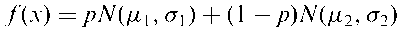where p is the proportion of the first group in the population, \xx, ox are, respectively, the mean and standard deviation of the variable in the first group and \x2, o2 are the corresponding values in the second group. An example of such a distribution is shown in Fig. 64. Mixtures of multivariate normal distributions are often used as models for cluster analysis. See also NORMIX, contaminated normal distribution, bimodality and multimodality. [MV2 Chapter 10.]

### Finite population:

A population of finite size.

### Finite population correction:

A term sometimes used to describe the extra factor in the variance of the sample mean when n sample values are drawn without replacement from a finite population of size N. This variance is given by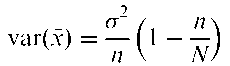the ‘correction’ term being (1 — n/N).

### First passage time:

An important concept in the theory of stochastic processes, being the time, T, until the first instant that a system enters a state j given that it starts in state i. Even for simple models of the underlying process very few analytical results are available for first passage times. [Fractals, 2000, 8, 139-45.]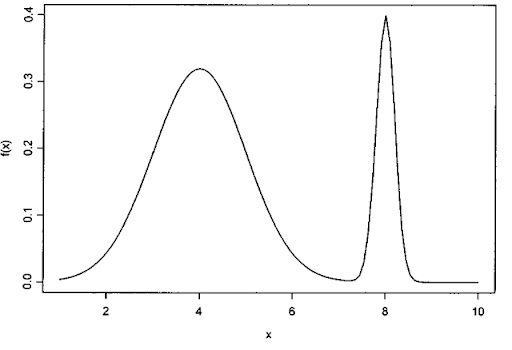Fig. 64 Finite mixture distribution with two normal components.

### Fisher, Irving (1867-1947):

Born in Saugerties, New York, Fisher studied mathematics at Yale, where he became Professor of Economics in 1895. Most remembered for his work on index numbers, he was also an early user of correlation and regression. Fisher was President of the American Statistical Association in 1932. He died on 29 April 1947 in New York.

### Fisher’s exact test:

An alternative procedure to use of the chi-squared statistic for assessing the independence of two variables forming a two-by-two contingency table particularly when the expected frequencies are small. The method consists of evaluating the sum of the probabilities associated with the observed table and all possible two-by-two tables that have the same row and column totals as the observed data but exhibit more extreme departure from independence. The probability of each table is calculated from the hypergeometric distribution.

### Fisher’s g statistic:

A statistic for assessing ordinates in a periodogram and given by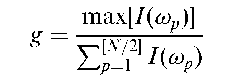where N is the length of the associated time series, and I(!p) is an ordinate of the periodogram. Under the hypothesis that the population ordinate is zero, the exact distribution of g can be derived. [Applications of Time Series Analysis in Astronomy and Meteorology, 1997, edited by T. Subba Rao, M.B. Priestley and O. Lissi, Chapman and Hall/CRC Press, London.]

### Fisher’s information:

Essentially the amount of information supplied by data about an unknown parameter. Given by the quantity;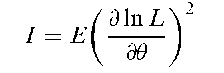where L is the likelihood of a sample of n independent observations from a probability distribution, f (x\0). In the case of a vector parameter, 9′ = [01,..., 0s], Fisher’s information matrix has elements j given by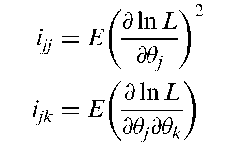The variance-covariance matrix of the maximum likelihood estimator of 9 is the inverse of this matrix. [KA2 Chapter 18.]

### Fisher’s linear discriminant function:

A technique for classifying an object or indivdual into one of two possible groups, on the basis of a set of measurements x1, x2,…, xq. The essence of the approach is to seek a linear transformation, z, of the measurements, i.e.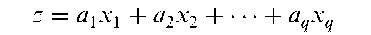such that the ratio of the between-group variance of z to its within-group variance is maximized. The solution for the coefficients a’ = [ai,..., aq] is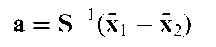where S is the pooled within groups variance-covariance matrix of the two groups and x 1, x2 are the group mean vectors.

### Fisher’s scoring method:

An alternative to the Newton-Raphson method for minimization of some function, which involves replacing the matrix of second derivatives of the function with respect to the parameters, by the corresponding matrix of expected values. This method will often converge from starting values further away from the minimum than will Newton-Raphson.

### Fisher, Sir Ronald Aylmer (1890-1962):

Born in East Finchley in Greater London, Fisher studied mathematics at Cambridge and became a statistician at the Rothamsted Agricultural Research Institute in 1919. By this time Fisher had already published papers introducing maximum likelihood estimation (although not yet by that name) and deriving the general sampling distribution of the correlation coefficient. At Rothamsted his work on data obtained in on-going field trials made him aware of the inadequacies of the arrangements of the experiments themselves and so led to the evolution of the science of experimental design and to the analysis of variance. From this work there emerged the crucial principle of randomization and one of the most important statistical texts of the 20th century, Statistical Methods for Research Workers, the first edition of which appeared in 1925 and now (1997) is in its ninth edition. Fisher continued to produce a succession of original research on a wide variety of statistical topics as well as on his related interests of genetics and evolutionary theory throughout his life. Perhaps Fisher’s central contribution to 20th century science was his deepening of the understanding of uncertainty and of the many types of measurable uncertainty. He was Professor of Eugenics at University College, London from 1933 until 1943, and then became the Arthur Balfour Professor of Genetics at Cambridge University. After his retirement in 1957, Fisher moved to Australia. He died on 29 July 1962 in Adelaide.

### Fisher’s z transformation:

A transformation of Pearson’s product moment correlation coefficient, r, given byThe statistic z has a normal distribution with mean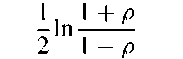where p is the population correlation value and variance 1 /(n — 3) where n is the sample size. The transformation may be used to test hypotheses and to construct confidence intervals for p. [SMR Chapter 11.]

### Fishing expedition:

Synonym for data dredging.

### Fitted value:

Usually used to refer to the value of the response variable as predicted by some estimated model.

### Five-number summary:

A method of summarizing a set of observations using the minimum value, the lower quartile, the median, upper quartile and maximum value. Forms the basis of the box-and-whisker plot.

### Fixed effects:

The effects attributable to a finite set of levels of a factor that are of specific interest. For example, the investigator may wish to compare the effects of three particular drugs on a response variable. Fixed effects models are those that contain only factors with this type of effect. See also random effects model and mixed effects models.

### Fixed population:

A group of individuals defined by a common fixed characteristic, such as all men born in Essex in 1944. Membership of such a population does not change over time by immigration or emigration.

### Fix-Neyman process:

A stochastic model used to describe recovery, relapse, death and loss of patients in medical follow-up studies of cancer patients.

### Fleiss, Joseph (1937-2003):

Born in Brooklyn, New York, Fleiss studied at Columbia College, graduating in 1959. In 1961 he received an M.S. degree in Biostatistics from the Columbia School of Public Health, and six years later completed his Ph.D. dissertation on ‘Analysis of variance in assessing errors in interview data’. In 1975 Fleiss became Professor and Head of the Division of Biostatistics at the School of Public Health in Columbia University. He made influential contributions in the analysis of categorical data and clinical trials and wrote two classic biosta-tistical texts, Statistical Methods for Rates and Proportions and The Design and Analysis of Clinical Experiments. Fleiss died on 12 June 2003, in Ridgewood, New Jersey.

### Flingner-Policello test:

A robust rank test for the Behrens-Fisher problem.

### Fligner-Wolfe test:

A distribution free method designed for the setting where one of the treatments in a study corresponds to a control or baseline set of conditions and interest centres on assessing which, if any, of the treatments are better than the control. The test statistic arises from combining all N observations from the k groups and ordering them from least to greatest. If r j is the rank of the jth observation in the ith group the test statistic is given specifically by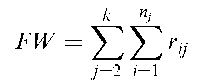the summations being over the non-control groups. Critical values of FW are available in appropriate tables, and a large-sample approximation can also be found.

### Flow-chart:

A graphical display illustrating the interrelationships between the different components of a system. It acts as a convenient bridge between the conceptualization of a model and the construction of equations.

### Folded log:

A term sometimes used for half the logistic transformation of a proportion.

### Folded normal distribution:

The probability distribution of Z = \X\, where the random variable, X, has a normal distribution with zero mean and variance a2. Given specifically byFolded square root: A term sometimes used for the following transformation of a proportion p

v2> – ffi -p)

### Foldover design:

A design for N points that consists of N/2 foldover pairs.

### Foldover frequency:

Synonym for Nyquist frequency.

### Foldover pair:

Two design points that are complementary in the sense that one is obtained by changing all the levels of all the factors in the other.

### Flog:

An unattractive synonym for folded log.

### Focus groups:

A research technique used to collect data through group interaction on a topic determined by the researcher. The goal in such groups is to learn the opinions and values of those involved with products and/or services from diverse points of view.

### Folk theorem of queueing behaviour:

The queue you join moves the slowest.

### Followback surveys:

Surveys which use lists associated with vital statistics to sample individuals for further information. For example, the 1988 National Mortality Followback Survey sampled death certificates for 1986 decedents 25 years or older that were filed in the USA. Information was then sought from the next of kin or some other person familiar with the decedent, and from health care facilities used by the decedent in the last year of life. Information was obtained by emailed questionnaire, or by telephone or personal interview. Another example of such a survey is the 1988 National Maternal and Infant Health Survey, the live birth component of which sampled birth certificates of live births in the USA. Mothers corresponding to the sampled birth certificates were then mailed a questionnaire. The sample designs of this type of survey are usually quite simple, involving a stratified selection of individuals from a list frame. [American Journal of Industrial Medicine, 1995, 27, 195-205.]

### Follow-up:

The process of locating research subjects or patients to determine whether or not some outcome of interest has occurred.

### Follow-up plots:

Plots for following up the frequency and patterns of longitudinal data. In one version the vertical axis consists of the time units of the study. Each subject is represented by a column, which is a vertical line proportional in length to the subject’s total time in the system.

### Force of mortality:

Synonym for hazard function.

### Forecast:

The specific projection that an investigator believes is most likely to provide an accurate prediction of a future value of some process. Generally used in the context of the analysis of time series. Many different methods of forecasting are available, for example autoregressive integrated moving average models.

### Forest plot:

A name sometimes given to a type of diagram commonly used in meta-analysis, in which point estimates and confidence intervals are displayed for all studies included in the analysis. An example from a meta-analysis of clozapine v other drugs in the treatment of schizophrenia is shown in Fig. 65. A drawback of such a plot is that the viewer’s eyes are often drawn to the least significant studies, because these have the widest confidence intervals and are graphically more imposing. [Methods in Meta-Analysis, 2000, A.J. Sutton, K.R. Abrams, D.R. Jones, T.A. Sheldon, and F. Song, Wiley, Chichester, UK.]

### Forward-looking study:

An alternative term for prospective study.

### Forward selection procedure:

See selection methods in regression.

### Fourfold table:

Synonym for two-by-two contingency table.

### Fourier series:

A series used in the analysis of generally a periodic function into its constituent sine waves of different frequencies and amplitudes. The series is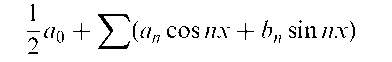where the coefficients are chosen so that the series converges to the function of interest, f; these coefficients (the Fourier coefficients) are given by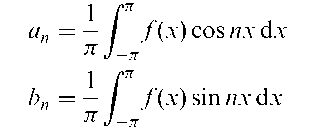for n = 1, 2, 3,…. See also fast Fourier transformation and wavelet analysis. [TMS Chapter 7.]

### Fractal:

A term used to describe a geometrical object that continues to exhibit detailed structure over a large range of scales. Snowflakes and coastlines are frequently quoted examples. A medical example is provided by electrocardiograms.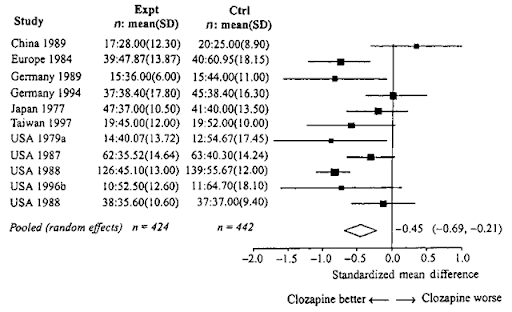Fig. 65 Forest plot.

### Fractal dimension:

A numerical measure of the degree of roughness of a fractal. Need not be a whole number, for example, the value for a typical coastline is between 1.15 and 1.25.

### Fractional factorial design:

Designs in which information on main effects and low-order interactions are obtained by running only a fraction of the complete factorial experiment and assuming that particular high-order interactions are negligible. Among the most widely used type of designs in industry. See also response surface methodology.

### Fractional polynomials:

An extended family of curves which are often more useful than low-or high-order polynomials for modelling the often curved relationship between a response variable and one or more continuous covariates. Formally such a polynomial of degree m is defined as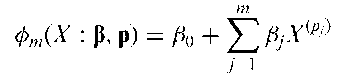where p’ = [p1,..., pm] is a real-valued vector of powers with p1 < p2 < ■■■ < pm and P’ = [/S0,..., pm] are real-valued coefficients. The round bracket notation signifies the Box-Tidwell transformation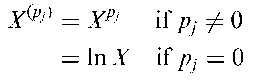So, for example, a fractional polynomial of degree 3 with powers (1,2,0) is of the form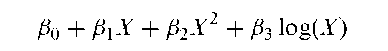### Frailty:

A term generally used for unobserved individual heterogeneity. Such variation is of major concern in medical statistics particularly in the analysis of survival times where hazard functions can be strongly influenced by selection effects operating in the population. There are a number of possible sources of this heterogeneity, the most obvious of which is that it reflects biological differences, so that, for example, some individuals are born with a weaker heart, or a genetic disposition for cancer. A further possibility is that the heterogeneity arises from the induced weaknesses that result from the stresses of life. Failure to take account of this type of variation may often obscure comparisons between groups, for example, by measures of relative risk. A simple model (frailty model) which attempts to allow for the variation between individuals is individual hazard function = ZX(t) where Z is a quantity specific to an individual, considered as a random variable over the population of individuals, and X(t) is a basic rate. What is observed in a population for which such a model holds is not the individual hazard rate but the net result for a number of individuals with different values of Z.

### Frank’s family of bivariate distributions:

A class of bivariate probability distributions of the form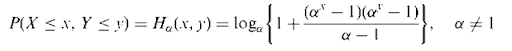A perspective plot of such a distribution is shown m Fig. 66.

### Freeman-Tukey test:

A procedure for assessing the goodness-of-fit of some model for a set of data involving counts. The test statistic is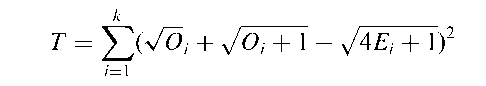where k is the number of categories, Oi, i = 1, 2,…, k the observed counts and Ei, i = 1, 2,…, k, the expected frequencies under the assumed model. The statistic T has asymptotically a chi-squared distribution with k — s — 1 degrees of freedom, where s is the number of parameters in the model. See also chi-squared statistic, and likelihood ratio.

### Freeman-Tukey transformation:

A transformation of a random variable, X, having a Poisson distribution, to the form Jx + VXT 1 in order to stabilize its variance.

### Frequency distribution:

The division of a sample of observations into a number of classes, together with the number of observations in each class. Acts as a useful summary of the main features of the data such as location, shape and spread. Essentially the empirical equivalent of the probability distribution. An example is as follows: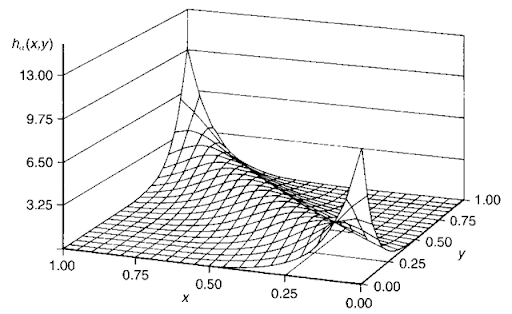Fig. 66 An example of a bivariate distribution from Frank’s family.

 Hormone assay values(nmol/L) Class limits Observed frequency 75-79 1 80-84 2 85-89 5 90-94 9 95-99 10 100-104 7 105-109 4 110-114 2 > 115 1

### Frequency polygon:

A diagram used to display graphically the values in a frequency distribution. The frequencies are graphed as ordinate against the class mid-points as abscissae. The points are then joined by a series of straight lines. Particularly useful in displaying a number of frequency distributions on the same diagram. An example is given in Fig. 67.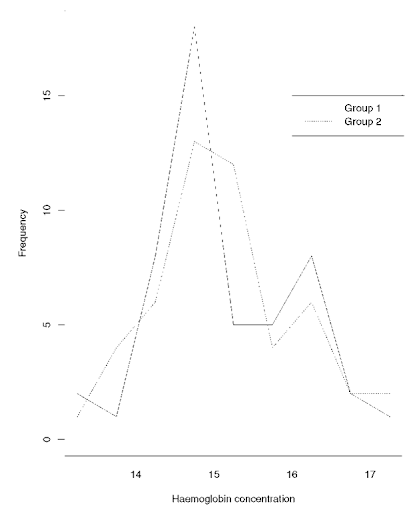Fig. 67 Frequency polygon of haemoglobin concentrates for two groups of men.

### Frequentist inference:

An approach to statistics based on a frequency view of probability in which it is assumed that it is possible to consider an infinite sequence of independent repetitions of the same statistical experiment. Significance tests, hypothesis tests and likelihood are the main tools associated with this form of inference. See also Bayesian inference.

### Friedman’s two-way analysis of variance:

A distribution free method that is the analogue of the analysis of variance for a design with two factors. Can be applied to data sets that do not meet the assumptions of the parametric approach, namely normality and homogeneity of variance. Uses only the ranks of the observations.

### Friedman’s urn model:

A possible alternative to random allocation of patients to treatments in a clinical trial with K treatments, that avoids the possible problem of imbalance when the number of available subjects is small. The model considers an urn containing balls of K different colours, and begins with w balls of colour k, k = 1,…, K .A draw consists of the following operations

• select a ball at random from the urn,

• notice its colour k’ and return the ball to the urn;

• add to the urn a more balls of colour k’ and f more balls of each other colour k where k = k’.

Each time a subject is waiting to be assigned to a treatment, a ball is drawn at random from the urn; if its colour is k’ then treatment k’ is assigned. The values of w, a and f can be any reasonable nonnegative numbers. If f is large with respect to a then the scheme forces the trial to be balanced. The value of w determines the first few stages of the trial. If w is large, more randomness is introduced into the trial; otherwise more balance is enforced.

### Froot:

An unattractive synonym for folded square root.

### F-test:

A test for the equality of the variances of two populations having normal distributions, based on the ratio of the variances of a sample of observations taken from each. Most often encountered in the analysis of variance, where testing whether particular variances are the same also tests for the equality of a set of means. [SMR Chapter 9.]

### Full model:

Synonym for saturated model.

### Functional data analysis:

The analysis of data that are functions observed continuously, for example, functions of time. Essentially a collection of statistical techniques for answering questions like ‘in what way do the curves in the sample differ?’, using information on the curves such as slopes and curvature.

### Functional principal components analysis:

A version of principal components analysis for data that may be considered as curves rather than the vectors of classical multi-variate analysis. Denoting the observations X1(t), X2 (t),…, Xn(t), where X,(t) is essentially a time series for individual i, the model assumed is that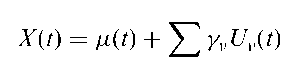where the principal component scores, yv are uncorrelated variables with mean zero, and the principal component functions, Uv(t) are scaled to satisfy f = 1; these functions often have interesting physical meanings which aid in the interpretation of the data.

### Functional relationship:

The relationship between the ‘true’ values of variables, i.e. the values assuming that the variables were measured without error. See also latent variables and structural equation models.

### Funnel plot:

An informal method of assessing the effect of publication bias, usually in the context of a meta-analysis. The effect measures from each reported study are plotted on the x-axis against the corresponding sample sizes on the y-axis. Because of the nature of sampling variability this plot should, in the absence of publication bias, have the shape of a pyramid with a tapering ‘funnel-like’ peak. Publication bias will tend to skew the pyramid by selectively excluding studies with small or no significant effects. Such studies predominate when the sample sizes are small but are increasingly less common as the sample sizes increase. Therefore their absence removes part of the lower left-hand corner of the pyramid. This effect is illustrated in Fig. 68.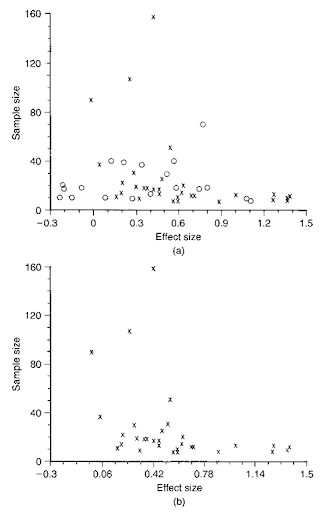Fig. 68 Funnel plot of studies of psychoeducational progress for surgical patients: (a) all studies; (b) published studies only.

### FU-plots:

Abbreviation for follow-up plots.

### Future years of life lost:

An alternative way of presenting data on mortality in a population, by using the difference between age at death and life expectancy. [An Introduction to Epidemiology, 1983, M.A. Alderson, Macmillan, London.]

### Fuzzy set theory:

A radically different approach to dealing with uncertainty than the traditional probabilistic and statistical methods. The essential feature of a fuzzy set is a membership function that assigns a grade of membership between 0 and 1 to each member of the set. Mathematically a membership function of a fuzzy set A is a mapping from a space x to the unit interval mA : x ! [0,1]. Because memberships take their values in the unit interval, it is tempting to think of them as probabilities; however, memberships do not follow the laws of probability and it is possible to allow an object to simultaneously hold nonzero degrees of membership in sets traditionally considered mutually exclusive. Methods derived from the theory have been proposed as alternatives to traditional statistical methods in areas such as quality control, linear regression and forecasting, although they have not met with universal acceptance and a number of statisticians have commented that they have found no solution using such an approach that could not have been achieved as least as effectively using probability and statistics. See also grade of membership model.

Previous post: